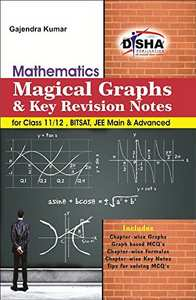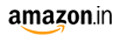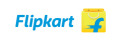# Magical Graphs and Key Revision Notes for Mathematics Class 11/12, BITSAT, JEE Main & Advanced JEE MainM.R.P 250/-119.00(52%)149.00(40%)
Product Description

This is a unique book that comprises all mathematical formulae at one place along with the study of all variety of Graphs encompassing the mathematics syllabus. The 1st part book covers 27 chapters just on formulae. The author has left no stone unturned in covering the exhaustive list of formulae. The second part of the book focuses on the concepts and numerical problems on Graphs. All the graphs have been covered and possible questions have been discussed. With 10 chapters on the concepts and numerical of Graphs, along with detailed graphical solutions, the book will not only help the young Engineering and Olympiad aspirants but shall also appeal to the Mathematics lovers and mentors of Engineering entrance examinations all over the country. It has been often found that many students struggle with formulae in Mathematics. On the other hand, there have been innumerable instances where just knowing a formula has triggered solution for a complex problem just in the blink of an eye. The book aims at a thorough understanding of the fundamentals of Graphs and learning useful problem solving techniques that will definitely see a candidate sail through tougher questions. A quick glimpse on the pattern of questions asked in IIT JEE and other leading exams like BITSAT, VITEEE, etc. will clearly show how tricky questions can become a problem if one is not well adept with the concept of Graphs. The book will be of immense help for all those students who are aiming for top ranks in various Engineering entrance examinations provided they solve each and every sum and go through all the formulae.

Part A – Graphs

• Straight Lines & Transformation of Graph
• Modulas
• Cubic and Polynomial Functions
• Piecewise Defined Function
• Rational Functions
• Basic Trigonometrical Function
• Inverse Trigonometrical Function
• Logarithmic & Exponential Functions
• Miscellaneous

Part B - Key Concepts & Formula

• Algebraic Formulae and Inequality
• Sequence and Series
• Logarithm
• Binomial Theorem
• Complex Numbers
• Determinants
• Matrices
• Permutation and Combination
• Probability
• Trigonometric Identity
• Trigonometric Equation and Inverse
• Properties of Triangles
• Straight Lines
• Circles
• Parabola
• Ellipse
• Hyperbola
• Functions & Relations
• Limit Continuity & Differentiability
• Differentiation
• Application of Derivative
• Indefinite Integration
• Definite Integration
• Differential Equation
• Vector Algebra
• Three Dimensional Geometry